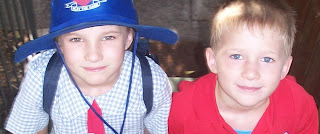## Thursday, February 15, 2007

### The Amazing Brain 2: A different right answer??

Here's another puzzle:

Someone has two children. One of them's a girl. (sound like you've already heard it?)

What's the probability the other one is a girl?

How about this answer: there are four possible combinations of boys and girls: BB, BG, GB, and GG. Now we know one of them is a girl, so that leaves three possible combinations, BG, GB, and GG, so the answer is one in three, or 33%.

Hold on, you say... well, I say it could be any of those combinations, and I didn't say which one of the two was the girl. Look at it this way. In an average population, there will be each of those combinations in roughly equal measure. So if you rule out BB, one third of the time the other child will be a girl.

So, bearing in mind yesterday's answer, how can you have two different answers at the same time?In fact, it really depends how you ask the question. The above question wasn't really phrased precisely enough at the start.

The pivotal issue is whether you're talking about probability, or sampling from a population. They're not exactly the same thing. The two puzzles yesterday were one question on sampling, and one on probability. Which is why there are different answers. On any one toss of a coin, the probability of heads will be 50% (all other things being equal, of course). But if you have a population of four coin tosses where two are heads and two tails, and you take away one of the tails, any given coin left that you choose blindly will have a third chance of being the other tail.

Probability vs sampling from a population.

What's this got to do with the brain? More anon. I read yesterday's two puzzles in a book, and it gave the same answer to each (ie, one third). I knew the answer to the first was 50%, but when I revisited the book, I could see the reasoning. It actually took me a couple of train stops to reconcile the two competing, apparently correct, answers.

The point in the book was that statistics is not always intuitive. How true.